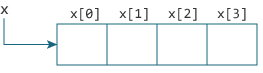Relationship Between Arrays and Pointers

# Relationship Between Arrays and Pointers

#### In this tutorial, you'll learn about the relationship between arrays and pointers in C programming. You will also learn to access array elements using pointers.

Before you learn about the relationship between arrays and pointers, be sure to check these two topics:

## Relationship Between Arrays and Pointers

An array is a block of sequential data. Let's write a program to print addresses of array elements.

``````#include <stdio.h>
int main() {
int x;
int i;

for(i = 0; i < 4; ++i) {
printf("&x[%d] = %p\n", i, &x[i]);
}

printf("Address of array x: %p", x);

return 0;
}
``````

Output

```&x = 1450734448
&x = 1450734452
&x = 1450734456
&x = 1450734460
```

There is a difference of 4 bytes between two consecutive elements of array x. It is because the size of `int` is 4 bytes (on our compiler).

Notice that, the address of &x and x is the same. It's because the variable name x points to the first element of the array.From the above example, it is clear that `&x` is equivalent to x. And, `x` is equivalent to `*x`.

Similarly,

• `&x` is equivalent to `x+1` and `x` is equivalent to `*(x+1)`.
• `&x` is equivalent to `x+2` and `x` is equivalent to `*(x+2)`.
• ...
• Basically, `&x[i]` is equivalent to `x+i` and `x[i]` is equivalent to `*(x+i)`.

### Example 1: Pointers and Arrays

``````#include <stdio.h>
int main() {
int i, x, sum = 0;
printf("Enter 6 numbers: ");
for(i = 0; i < 6; ++i) {
// Equivalent to scanf("%d", &x[i]);
scanf("%d", x+i);

// Equivalent to sum += x[i]
sum += *(x+i);
}
printf("Sum = %d", sum);
return 0;
}
``````

When you run the program, the output will be:

```Enter 6 numbers:  2
3
4
4
12
4
Sum = 29 ```

Here, we have declared an array x of 6 elements. To access elements of the array, we have used pointers.

In most contexts, array names decay to pointers. In simple words, array names are converted to pointers. That's the reason why you can use pointers to access elements of arrays. However, you should remember that pointers and arrays are not the same.

There are a few cases where array names don't decay to pointers. To learn more, visit: When does array name doesn't decay into a pointer?

### Example 2: Arrays and Pointers

``````#include <stdio.h>
int main() {
int x = {1, 2, 3, 4, 5};
int* ptr;

// ptr is assigned the address of the third element
ptr = &x;

printf("*ptr = %d \n", *ptr);   // 3
printf("*(ptr+1) = %d \n", *(ptr+1)); // 4
printf("*(ptr-1) = %d", *(ptr-1));  // 2

return 0;
}``````

When you run the program, the output will be:

```*ptr = 3
*(ptr+1) = 4
*(ptr-1) = 2```

In this example, `&x`, the address of the third element, is assigned to the ptr pointer. Hence, `3` was displayed when we printed `*ptr`.

And, printing `*(ptr+1)` gives us the fourth element. Similarly, printing `*(ptr-1)` gives us the second element.